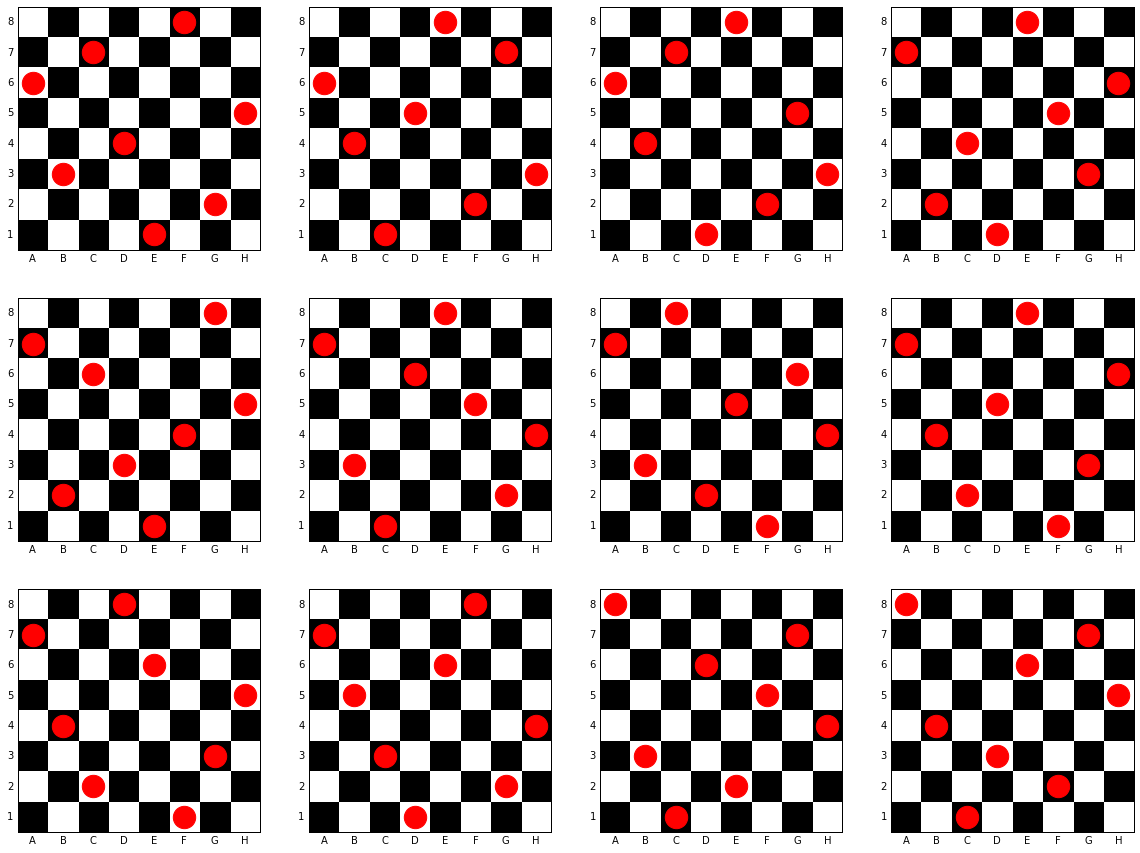# Solving the 8 Queens problem with python

Posted on Mon 28 March 2016 in Problem solving

This is my approach to solving the 8 Queens puzzle with Python.

For anyone unfamiliar with the 8 Queens puzzle, it is the problem of placing eight queens on a standard (8x8) chessboard such that no queen is in a position that can attack any other. This post will have the solutions to the puzzle, so if you’d like to attempt to solve it on your own, now would be a good time to stop reading this post.

I was first made aware of the existence of this puzzle in a pub one evening with some friends. One of my friends started trying to solve the puzzle manually and found a solution in about 10 minutes. This inspired me to attempt to tackle the problem with Python to see if I would have been able to find a solution faster. I took me around 15 minutes to solve the puzzle using python, but found 92 solutions (there are 12 if you eliminate symmetrically related solutions).

This original code I wrote to solve the problem looked like this:

```from itertools import permutations, combinations

text = input('How big is your chess board?')
n = int(text)
x = range(1, n+1)

def is_diagonal(point1, point2):
x1 = point1
y1 = point1
x2 = point2
y2 = point2
return(True)
else:
return(False)

list_of_permutations = []

for permuation in permutations(range(1, n+1)):
y = permuation
all_permutations = list(zip(x,y))
list_of_permutations.append(all_permutations)

for possible_solution in list_of_permutations:
solutions = []
for piece1, piece2 in combinations(possible_solution, 2):
solutions.append(is_diagonal(piece1, piece2))

if True not in solutions:
print(possible_solution)
```

I’ve since expanded it to make it easier to understand, abstracting some useful functions and added some code to remove equivalent solutions and help visualise the solutions, but the code above contains the main logic that runs at the heart of the approach I took. The expanded version of the code can be found here.

Let’s break it down a little bit to explain what’s happening.

We know that no two queens can attack each other. This means that there must be 1 queen per row. Similarly, there must be 1 queen per column. In this approach, we’re going to take 8 queens, assign them to the rows 1-8 and determine what columns they must each be in in order for the puzzle criteria to be satisfied. Since there are 8 queens and 8 column positions, there are 40,320 (nPr with n=r=8) ways to arrange 8 queens on a chessboard such that there is one queen per row and 1 queen per column. Since we already know what none of the queens will be attacking any other horizontally or vertically, all we need to do is to check each of the 40,320 arrangements to see if any queen is diagonally threatening any other. This takes about a second to run in total (1.06 seconds on my mid-range 5-year-old Desktop computer) for all 40,320 possible queen arrangements and returns 92 solutions that fit the criteria of having no queen attacking any other. Some of these will be symmetrically related. For example, here are 8 solutions from the set of 92 that are related to each other through 90 or 180 degree rotations; or mirror planes (i.e. they are horizontal, vertical or diagonal mirror images of each other).When we remove the solutions that are related, we are left with the 12 unique solutions for the 8x8 board case, shown below:The Jupyter notebook containing the current version of the code is available here

Thanks to my friends:

• Daniele Tomerini for introducing me to this puzzle
• Hugh Thompson, whose attempts at solving this puzzle manually inspired me to tackle it using python